Courses

# Thermal Runaway And Thermal Stability Electrical Engineering (EE) Notes | EduRev

## Electronic Devices

Created by: Cstoppers Instructors

## Electrical Engineering (EE) : Thermal Runaway And Thermal Stability Electrical Engineering (EE) Notes | EduRev

The document Thermal Runaway And Thermal Stability Electrical Engineering (EE) Notes | EduRev is a part of the Electrical Engineering (EE) Course Electronic Devices.
All you need of Electrical Engineering (EE) at this link: Electrical Engineering (EE)

THERMAL RUNAWAY:

The collector current for the CE circuit is given by IC = βIB+(1+β)ICO . The three variables in the equation, β, IB, and ICO increase with rise in temperature. In particular, the reverse saturation current or leakage current ICO changes greatly with temperature. Specifically, it doubles for every 10oC rise in temperature. The collector current Icauses the collector base junction temperature to rise which in turn, increase ICO, as a result IC will increase still further, which will further increase the temperature at the collector base junction. This process will become cumulative at the collector base junction leading to “thermal runaway”. Consequently, the ratings of the transistor are exceeded which may destroy the transistor itself.

The collector is made larger in size than the emitter in order to facilitate the heat dissipation at the collector junction. However if the circuit is designed such that the base current Iis made to decrease automatically with rise in temperature, then the decrease in βIB will compensate for increase in the (1+β)ICO, keeping IC almost constant.

THERMAL RESISTANCE

Consider transistor used in a circuit where the ambient temperature of the air around the transistor is TAoC and the temperature of the collector-base junction of the transistor is TJoC.

Due to heating within the transistor, TJ is higher than TA. As the temperature difference TJ- TA is greater, the power dissipated in the transistor, PD will be greater, i.e,

(TJ- TA) ∝ PD

The equation can be written as TJ- T=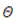PD, whereis the constant of proportionality and is called the Thermal resistance. Rearranging the above equation= (TJ- TA) /PD. Henceis measured in oC/W which may be as small as 0.2 oC/W for a high power transistor that has an efficient heat sink of up to 1000oC/W for small signal, low power transistor which have no cooling provision.

As Θ represents total thermal resistance from a transistor junction to the ambient temperature, it is referred to as ΘJ-A.  However, for power transistors, thermal resistance is given as ΘJ-C.

The amount of resistance from junction to ambience is considered to consist of 2 parts.

ΘJ-A = ΘJ-C - ΘC-A.

which indicates that the heat dissipated in the junction must make its way to the surrounding air through two series paths from junction to case and from case to air. Hence the power dissipated is given as-

PD = (TJ- TA) / Θ J-A

=(TJ- TA )/ Θ J-C + Θ C-A)

ΘJ-C is determined by the type of manufacturer of the transistor and how it is located in the case, but ΘC-A is determined by the surface area of the case or flange and its contact with air. If the effective surface area of the transistor case could be increased, the resistance to heat flows could be increased ΘC-A, could be decreased. This can be achieved by the use of a heat sink.

The heat sink is a relatively large, finned, usually black metallic heat conducting device in close contact with transistor case or flange. Many versions of heat sink exist depending upon the shape and size of the transistor. Larger the heat sink smaller is the thermal resistance ΘHS-A.

This thermal resistance is not added to ΘC-A in series, but is instead in parallel with it and if ΘHS-A is much less than ΘC-A, then ΘC-A will be reduced significantly, thereby improving the dissipation capability of the transistor. Thus

Θ J-AJ-C + Θ C-A|| ΘHS-A.

4.8 CONDITION FOR THERMAL STABILITY:

For preventing thermal runaway, the required condition is the rate at which the heat is released at the collector junction should not exceed the rate at which the heat can be dissipated under steady state conditions. Hence the condition to be satisfied to avoid thermal runaway is given by-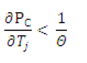If the circuit is properly designed, then the transistor cannot runaway below a specified ambient temperature  or even under any condition.

In the self biased circuit the transistor is biased in the active region. The power generated at the junction without any signal is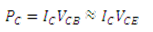Let us assume that the quiescent collector and the emitter currents are equal. Then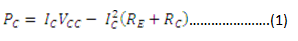The condition to prevent thermal runaway can be written as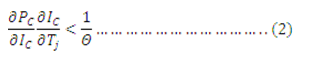As Θ and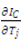are positive,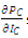should be negative in order to satisfy the above condition.

Differentiating  equation  (1) w.r.t  Iwe get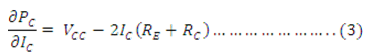Hence to avoid thermal runaway it is necessary that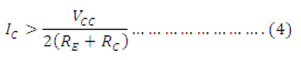SinceVCE=VCC-IC(RE+RC) then eq (4) implies that VCE<VCC/2. If the inequality of eq (4) is not satisfied and VCE<VCC/2, then from eq(3),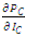is positive., and the corresponding eq(2) should be satisfied. Other wise thermal runaway will occur.

Offer running on EduRev: Apply code STAYHOME200 to get INR 200 off on our premium plan EduRev Infinity!

,

,

,

,

,

,

,

,

,

,

,

,

,

,

,

,

,

,

,

,

,

;